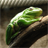# Using Raster Calculator in Model Builder

1801
3
03-16-2012 12:13 AMNew Contributor II
Hello, I have constructed a model that uses an OLS regression towards the end.  I would like the values for two of the coefficients to be used as part of a raster calculator equation that rebuilds the regression and outputs to a raster.  I have entered the equation that I want to use in the Raster Calculator tool, but cannot figure out how to make the two coefficients from the OLS regression become part of the equation (they are not listed as options under the Layers and Variables section of Raster Calculator).  Part of the problem is that the model is designed to have those two coefficients be different every time the model is run.  Is there any way that I can have the two coefficients become part of the equation?  I have attached a screen shot of the model for clarity.  Any help is greatly appreciated...

Thanks,

Graham
Tags (3)
3 RepliesNew Contributor II
Perhaps I should mention that I attached the Variables bubble to the Raster Calculator bubble with a precondition connector.  The other option was a Map Algebra Expression connector, but when I tried that no connector appeared on the model connecting the two bubbles.

Thanks,

GrahamNew Contributor IIby